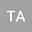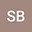loading page

Two Operator Boundary - Domain Integral Equations for variable coefficient Neumann BVP in 2D
••Solomon BEKELE
Addis Ababa University
Author Profile## Abstract

The Neumann boundary value problem (BVP) for the second order “stationary heat transfer” elliptic partial differential equation with variable coefficient is considered in two-dimensional bounded domain. Using an appropriate parametrix (Levi function) and applying the two-operator approach, this problem is reduced to some systems of boundary-domain integral equations (BDIEs). The two-operator BDIEs in 2D have special consideration due to their different equivalence properties as compared to higher dimensional case due to the logarithmic term in the parametrix for the associated partial differential equation. Consequently, we need to set conditions on the domain or function spaces to insure the invertibility of the corresponding layer potentials, and hence the unique solvability of BDIEs. Equivalence of the two operator BDIE systems to the original Neumann BVP, BDIEs solvability, uniqueness/non uniqueness of the solution, as well as Fredholm property and invertibility of the BDIE operator are analysed. Moreover, the two operator boundary domain integral operators for the Neumann BVP are not invertible, and appropriate finite-dimensional perturbations are constructed leading to invertibility of the perturbed operators.\\ \noindent\textbf{Key words:} Partial differential equation, Two-operator Boundary-Domain Integral Equations, finite-dimensional perturbations , equivalence, invertibility.